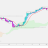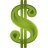# How to Use the TOS Scanner

J

##### New member
This is probably simple but I would like to scan for when the last candle was green preceded by red candles.

input agg = AggregationPeriod.WEEK;
input TrendPeriods = 6;

def o = open(period = agg);
def h = high(period = agg);
def l = low(period = agg);
def c = close(period = agg);
def hh = highest(h, trendPeriods);
def ll = lowest(l, trendPeriods);
def trend = if ((c - ll) / (hh - ll)) > .5
then 1
else 0;Questions 0Indicators 18